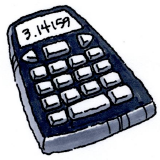### Home > CCAA8 > Chapter 8 Unit 9 > Lesson CCA: 8.2.3 > Problem8-88

8-88.

Calculate the value of each expression below using a scientific calculator.1. $\frac{-10+\sqrt{25}}{ 5}$

• Calculate the square root, then simplify the rest of the expression.

• $\frac{-10+5}{5}$

1. $\frac{8+\sqrt{40}}{ 3\cdot3}$

Calculate the square root and everything in the denominator before simplifying the rest of the expression.

1. $\frac{8+\sqrt{3^2+2\cdot3+1}}{-4}$

Calculate everything inside the square root before simplifying.